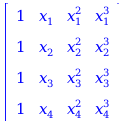Thermophysical Data - Maple Help

Home : Support : Online Help : System : Information : Updates : Maple 2018 : Thermophysical Data

Thermophysical Data and Scientific ConstantsIntroduction

The ThermophysicalData package

 • introduces the Chemicals subpackage, which gives the properties of an additional 2000 chemical species
 • and updates the CoolProp fluid properties engine to version 6.1

Additionally, the non-derived physical constants in the ScientificConstants package are updated to reflect those in the 2014 release of the CODATA Recommended Values of the Fundamental Physical Constants.New Thermodynamic Data

Maple 2018 introduces the Chemicals subpackage. This uses a new data source* to give the thermodynamic properties of over 2000 gases, liquid and crystalline species.

* Bonnie J. McBride, Michael J. Zehe, and Sanford Gordon. NASA Glenn Coefficients for Calculating Thermodynamic Properties of Individual Species; 2002; https://www.grc.nasa.gov/WWW/CEAWeb/TP-2002-21556.htm.

The data can be used to study

 • chemical equilibrium composition
 • reaction constants and spontaneity
 • rocket performance
 • flame temperatures
 • explosion and detonation pressures
 • and many more applications

 > $\mathrm{restart}:\phantom{\rule[-0.0ex]{0.0em}{0.0ex}}\phantom{\rule[-0.0ex]{0.0em}{0.0ex}}\mathrm{with}\left(\mathrm{ThermophysicalData}\right)$
 $\left[{\mathrm{Chemicals}}{,}{\mathrm{CoolProp}}{,}{\mathrm{PHTChart}}{,}{\mathrm{Property}}{,}{\mathrm{PsychrometricChart}}{,}{\mathrm{TemperatureEntropyChart}}\right]$ (2.1)

Heat of formation and molar mass of gaseous CO2

 > $\mathrm{Chemicals}:-\mathrm{Property}\left("HeatOfFormation","CO2\left(g\right)",\mathrm{useunits}\right);\phantom{\rule[-0.0ex]{0.0em}{0.0ex}}\phantom{\rule[-0.0ex]{0.0em}{0.0ex}}$$\mathrm{Chemicals}:-\mathrm{Property}\left("MolarMass","CO2\left(g\right)",\mathrm{useunits}\right);$
 ${-}{3.93510000}{}{{10}}^{{5}}{}⟦\frac{{J}}{{\mathrm{mol}}}⟧$
 ${44.0095000}{}⟦\frac{{g}}{{\mathrm{mol}}}⟧$ (2.2)

Enthalpy and entropy of gaseous CO2 at 300 K

 >
 ${-}{3.934412212}{}{{10}}^{{5}}{}⟦\frac{{J}}{{\mathrm{mol}}}⟧$
 ${214.0173732}{}⟦\frac{{J}}{{\mathrm{mol}}{}{K}}⟧$ (2.3)

See help for more detail, including a list of the species.Application: Adiabatic Flame Temperature of Butane

Liquid butane is burnt with 100% theoretical air at an initial temperature of 298.15 K. The combustion reaction is

C4H10 (l) + 6.5 O2 (g)+ 24.44 N2 (g) → 4 CO2 (g) + 5 H2O (g) + 24.44 N2 (g)

Here, we will calculate the adiabatic flame temperature of the combustion products.

Heat of formation of butane

 > $\mathrm{h_f_C4H10}≔\mathrm{Chemicals}:-\mathrm{Property}\left("HeatOfFormation","C4H10\left(l\right),n-buta",\mathrm{useunits}\right)$
 ${-}{150.6640000}{}⟦\frac{{\mathrm{kJ}}}{{\mathrm{mol}}}⟧$ (2.1.1)

Enthalpies of the combustion products at a temperature T

 > $\mathrm{h_N2}≔\mathrm{Chemicals}:-\mathrm{Property}\left("Hmolar","N2\left(g\right)","temperature"=\mathrm{T}\right):$$\phantom{\rule[-0.0ex]{0.0em}{0.0ex}}\mathrm{h_O2}≔\mathrm{Chemicals}:-\mathrm{Property}\left("Hmolar","O2\left(g\right)","temperature"=\mathrm{T}\right):\phantom{\rule[-0.0ex]{0.0em}{0.0ex}}\phantom{\rule[-0.0ex]{0.0em}{0.0ex}}$$\mathrm{h_H2O}≔\mathrm{Chemicals}:-\mathrm{Property}\left("Hmolar","H2O\left(g\right)","temperature"=\mathrm{T}\right):\phantom{\rule[-0.0ex]{0.0em}{0.0ex}}\phantom{\rule[-0.0ex]{0.0em}{0.0ex}}$$\mathrm{h_CO2}≔\mathrm{Chemicals}:-\mathrm{Property}\left("Hmolar","CO2\left(g\right)","temperature"=\mathrm{T}\right):$

Enthalpy of the reactants

 >
 ${-}{150.6640000}{}⟦{\mathrm{kJ}}⟧$ (2.1.2)

Total enthalpy of the combustion products

 >
 ${\mathrm{H_products}}{≔}{4}{}{\mathrm{Chemicals}}{:-}{\mathrm{Property}}{}\left({"Hmolar"}{,}{"CO2\left(g\right)"}{,}{"temperature"}{=}{T}\right){}⟦{\mathrm{mol}}⟧{+}{5}{}{\mathrm{Chemicals}}{:-}{\mathrm{Property}}{}\left({"Hmolar"}{,}{"H2O\left(g\right)"}{,}{"temperature"}{=}{T}\right){}⟦{\mathrm{mol}}⟧{+}{24.44}{}{\mathrm{Chemicals}}{:-}{\mathrm{Property}}{}\left({"Hmolar"}{,}{"N2\left(g\right)"}{,}{"temperature"}{=}{T}\right){}⟦{\mathrm{mol}}⟧$ (2.1.3)

Equating the enthalpy of the reactants and the enthalpy of the combustion products gives the adiabatic flame temperature

 > $\mathrm{fsolve}\left(\mathrm{H_reactants}=\mathrm{H_products},\mathrm{T}=2000⟦\mathrm{K}⟧\right)$
 ${2379.853026}{}⟦{K}⟧$ (2.1.4)Application: Equilibrium Composition of the Combustion of Carbon Monoxide and Oxygen

One mole of CO and 0.5 moles of O2 are burned at 3000 K

CO (g) + 0.5 O2 (g) → CO2 (g)

The combustion products undergo dissociation and contain CO2, CO, O and O2. Here, we will calculate the equilibrium composition of the combustion productsPhysical Properties

Enthalpies as a function of temperature

 > $\mathrm{h_CO}≔\mathrm{Chemicals}:-\mathrm{Property}\left("Hmolar","CO\left(g\right)","temperature"=\mathrm{T}\right):$$\phantom{\rule[-0.0ex]{0.0em}{0.0ex}}\phantom{\rule[-0.0ex]{0.0em}{0.0ex}}\mathrm{h_C}≔\mathrm{Chemicals}:-\mathrm{Property}\left("Hmolar","C\left(gr\right)","temperature"=\mathrm{T}\right):\phantom{\rule[-0.0ex]{0.0em}{0.0ex}}\phantom{\rule[-0.0ex]{0.0em}{0.0ex}}$$\mathrm{h_O2}≔\mathrm{Chemicals}:-\mathrm{Property}\left("Hmolar","O2\left(g\right)","temperature"=\mathrm{T}\right):\phantom{\rule[-0.0ex]{0.0em}{0.0ex}}\phantom{\rule[-0.0ex]{0.0em}{0.0ex}}$$\mathrm{h_O}≔\mathrm{Chemicals}:-\mathrm{Property}\left("Hmolar","O\left(g\right)","temperature"=\mathrm{T}\right):$$\phantom{\rule[-0.0ex]{0.0em}{0.0ex}}\phantom{\rule[-0.0ex]{0.0em}{0.0ex}}\mathrm{h_CO2}≔\mathrm{Chemicals}:-\mathrm{Property}\left("Hmolar","CO2\left(g\right)","temperature"=\mathrm{T}\right):$

Entropy as a function of temperature

 > $\mathrm{s_CO}≔\mathrm{Chemicals}:-\mathrm{Property}\left("Smolar","CO\left(g\right)","temperature"=\mathrm{T}\right):$$\mathrm{s_O2}≔\mathrm{Chemicals}:-\mathrm{Property}\left("Smolar","O2\left(g\right)","temperature"=\mathrm{T}\right):\phantom{\rule[-0.0ex]{0.0em}{0.0ex}}\phantom{\rule[-0.0ex]{0.0em}{0.0ex}}$$\mathrm{s_O}≔\mathrm{Chemicals}:-\mathrm{Property}\left("Smolar","O\left(g\right)","temperature"=\mathrm{T}\right):\phantom{\rule[-0.0ex]{0.0em}{0.0ex}}\phantom{\rule[-0.0ex]{0.0em}{0.0ex}}$$\mathrm{s_CO2}≔\mathrm{Chemicals}:-\mathrm{Property}\left("Smolar","CO2\left(g\right)","temperature"=\mathrm{T}\right):$

Gibbs Free Energy as a function of temperature

 >

Universal gas constant

 >Constraints

Balancing the reactants and products gives

CO + 0.5 O2 = n1 CO2 + n2 CO + n3 O + n4 O2

This results in the following constraint on the oxygen atoms...

 >

...and this constraint on the carbon atoms

 > 

Total number of moles in products

 > $\mathrm{nt}≔\mathrm{n1}+\mathrm{n2}+\mathrm{n3}+\mathrm{n4}:$Equilibrium Composition

For a given temperature, minimizing the Gibbs Free Energy of the combustion products will give the equilibrium molar composition

 > $\mathrm{gibbs}≔\mathrm{n1}\left(\mathrm{G_CO2}\left(\mathrm{T}\right)+\mathrm{R}\mathrm{T}\mathrm{ln}\left(\frac{\mathrm{n1}}{\mathrm{nt}}\right)\right)+\mathrm{n2}\left(\mathrm{G_CO}\left(\mathrm{T}\right)+\mathrm{R}\mathrm{T}\mathrm{ln}\left(\frac{\mathrm{n2}}{\mathrm{nt}}\right)\right)+\mathrm{n3}\left(\mathrm{G_O}\left(\mathrm{T}\right)+\mathrm{R}\mathrm{T}\mathrm{ln}\left(\frac{\mathrm{n3}}{\mathrm{nt}}\right)\right)+\mathrm{n4}\left(\mathrm{G_O2}\left(\mathrm{T}\right)+\mathrm{R}\mathrm{T}\mathrm{ln}\left(\frac{\mathrm{n4}}{\mathrm{nt}}\right)\right):$
 >
 $\left[{-}{4.16167796100690844}{}{{10}}^{{5}}{}⟦{J}⟧{,}\left[{\mathrm{n1}}{=}{0.545813996336248}{}⟦{\mathrm{mol}}⟧{,}{\mathrm{n2}}{=}{0.454186003663752}{}⟦{\mathrm{mol}}⟧{,}{\mathrm{n3}}{=}{0.0565244627353324}{}⟦{\mathrm{mol}}⟧{,}{\mathrm{n4}}{=}{0.198830770464210}{}⟦{\mathrm{mol}}⟧\right]\right]$ (2.2.3.1)Updated CoolProp Library

Maple 2018 updates the CoolProp library to version 6.1. This includes new fluids and updated routines used to calculate fluid properties.

New fluids include Dichloroethane, DiethylEther, EthyleneOxide, HydrogenChloride, Novec 649TM and several others.

 > $\mathrm{Property}\left("enthalpy","R245ca","temperature"=298⟦\mathrm{K}⟧,"pressure"=1⟦\mathrm{atm}⟧\right)$
 ${233.8629511}{}⟦\frac{{\mathrm{kJ}}}{{\mathrm{kg}}}⟧$ (3.1)
 >
 ${3.649016897}{}⟦{\mathrm{MPa}}⟧$ (3.2)Updated ScientificConstants Package

The non-derived physical constants in the ScientificConstants package now reflect the most recent values published by CODATA.

 > $\mathrm{with}\left(\mathrm{ScientificConstants}\right)$
 $\left[{\mathrm{AddConstant}}{,}{\mathrm{AddElement}}{,}{\mathrm{AddProperty}}{,}{\mathrm{Constant}}{,}{\mathrm{Element}}{,}{\mathrm{GetConstant}}{,}{\mathrm{GetConstants}}{,}{\mathrm{GetElement}}{,}{\mathrm{GetElements}}{,}{\mathrm{GetError}}{,}{\mathrm{GetIsotopes}}{,}{\mathrm{GetProperties}}{,}{\mathrm{GetProperty}}{,}{\mathrm{GetUnit}}{,}{\mathrm{GetValue}}{,}{\mathrm{HasConstant}}{,}{\mathrm{HasElement}}{,}{\mathrm{HasProperty}}{,}{\mathrm{ModifyConstant}}{,}{\mathrm{ModifyElement}}\right]$ (4.1)
 > $\mathrm{GetConstant}\left(G\right)$
 ${\mathrm{Newtonian_constant_of_gravitation}}{,}{\mathrm{symbol}}{=}{G}{,}{\mathrm{value}}{=}{6.67408}{}{{10}}^{{-11}}{,}{\mathrm{uncertainty}}{=}{3.1}{}{{10}}^{{-15}}{,}{\mathrm{units}}{=}\frac{{{m}}^{{3}}}{{\mathrm{kg}}{}{{s}}^{{2}}}$ (4.2)
 >

 ApplicationsTheoretical Rocket PerformanceRich and Lean Octane CombustionEquilibrium Composition and Flame Temperature of the Combustion of Carbon MonoxideDeflagration Pressure of the Combustion of Hydrogen in Air at Constant Volume